Function Repository Resource:

# ZolotarevZ

Evaluate the Zolotarev polynomial

Contributed by: Jan Mangaldan
 ResourceFunction["ZolotarevZ"][p,q,x,m] gives the Zolotarev polynomial Zp,q(x|m).

## Details

Mathematical function, suitable for both symbolic and numerical manipulation.
The Zolotarev polynomial Zp,q(x|m) with elliptic parameter m is the polynomial that least deviates from zero, such that it has p zeroes to the left of its global maximum in [-1,1] and q zeroes to the right of it.
Zp,q(x|m) is a polynomial of degree p+q.
For certain special arguments, ResourceFunction["ZolotarevZ"] automatically evaluates to exact values.
ResourceFunction["ZolotarevZ"] can be evaluated to arbitrary numerical precision.

## Examples

### Basic Examples (2)

Evaluate numerically:

 In:=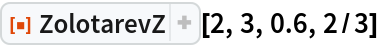Out=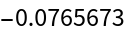Plot over a subset of the reals:

 In:=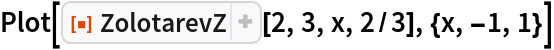Out=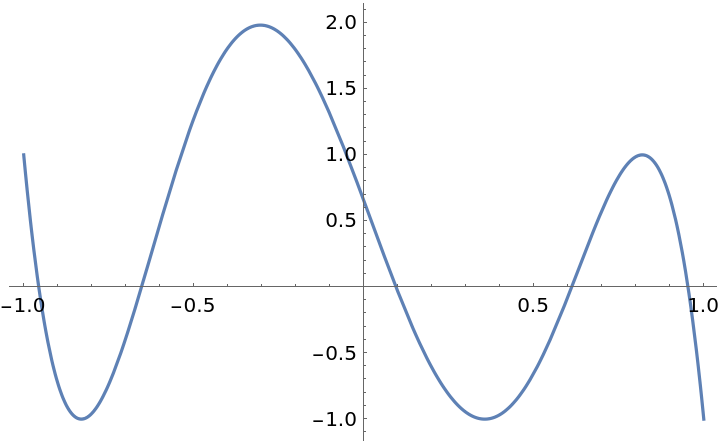### Scope (3)

Evaluate to high precision:

 In:=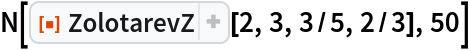Out=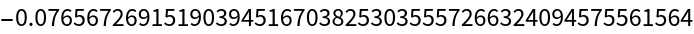Evaluate with symbolic argument and numerical parameter:

 In:=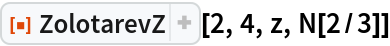Out=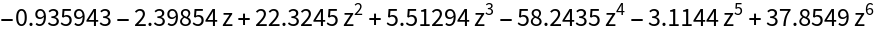In:=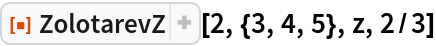Out=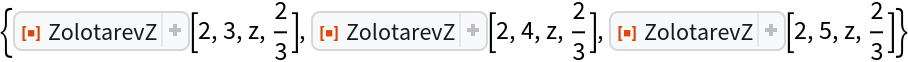### Applications (2)

Plot a Zolotarev polynomial and show the location of its global maximum in [-1,1]:

 In:=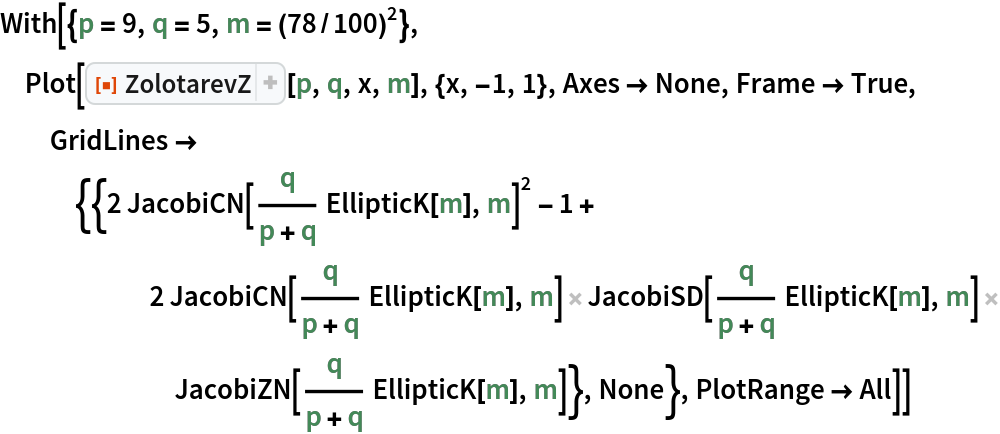Out=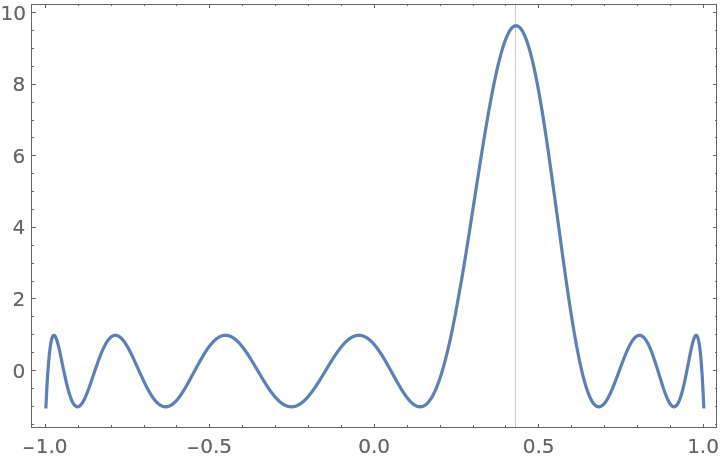Compare a Zolotarev polynomial with a Chebyshev polynomial of the same degree:

 In:=Out=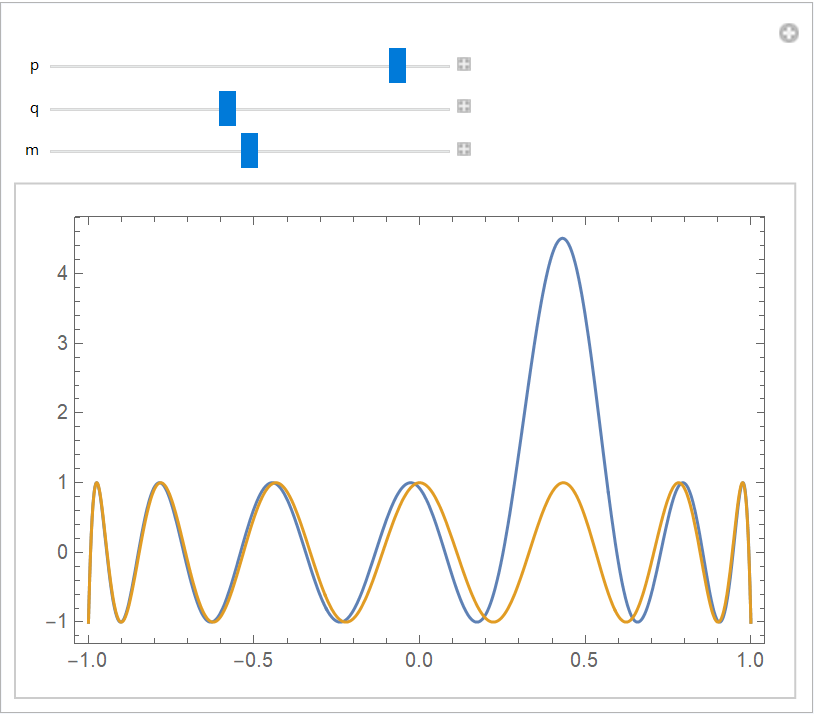### Properties and Relations (2)

When m=0 or m=1, the Zolotarev polynomial is a scalar multiple of the Chebyshev polynomial ChebyshevT:

 In:=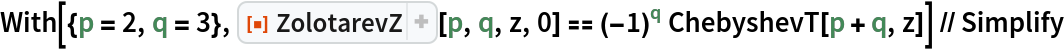Out=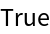In:=Out=A parametric representation of the Zolotarev polynomial:

 In:=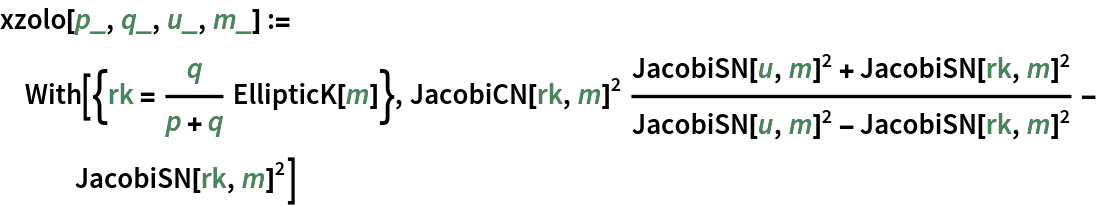In:=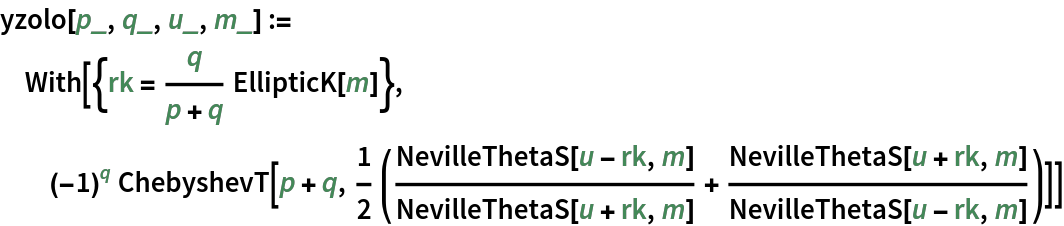Check equivalence with the explicit representation:

 In:=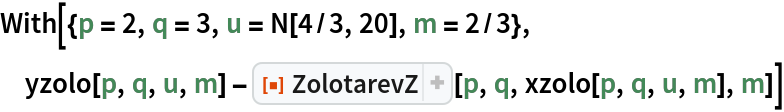Out=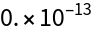### Possible Issues (1)

The literature sometimes uses a different definition of the Zolotarev polynomial, where the polynomial is defined to have p zeroes in [-1,1]:

 In:=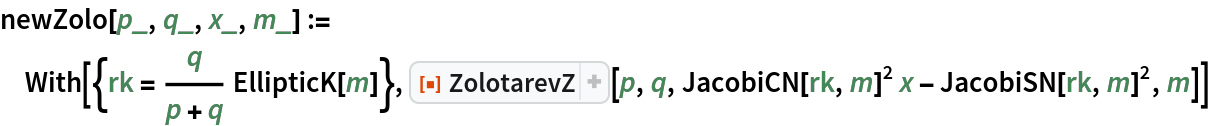In:=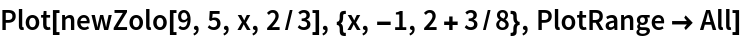Out=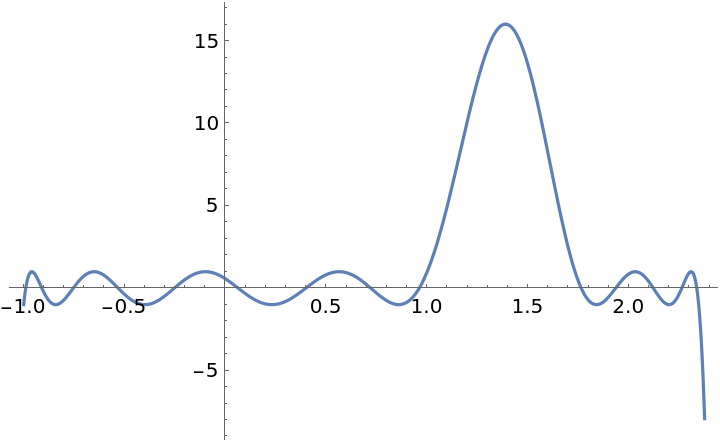## Version History

• 1.0.0 – 20 January 2021